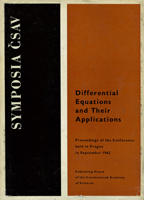Previous |  Up |  Next

## Differential Equations and Their Applications Proceedings of the Conference held in Prague in September 1962Editor:
Organized by: Czechoslovak Academy of Sciences; Association of Czechoslovak Mathematicians and Physicists
Venue: Prague, 1962

Publisher: Publishing House of the Czechoslovak Academy of Sciences, Prague, 1963

MR0167666 | Zbl 0128.00303
MSC: 00B25, 34-06, 35-06

 5 Preface.
 7 Contents.
 13-26 The theory of small changesin the domain of existence in the theory of partial differential equations and its applications.  Babuška, I.
 27-38 Transformation of ordinary second-order linear diferential equations.  Borůvka, O.
 39-54 Integral manifolds and nonlinear oscillations.  Hale, Jack K.
 55-63 Solutions of a linear second order equation of parabolic type defined in an unbounded domain.  Krzyżański, M.
 65-76 Problems which lead to a generalization of the concept of an ordinary nonlinear differential equation.  Kurzweil, J.
 77-92 Variational methods of solving linear and nonlinear boundary value problems.  Mihlin, S. G.
 93-104 On the solution of elliptic partial differential equations with an unbounded Dirichlet integral.  Nečas, J.
 105-120 On some qualitative methods for systems of ordinary diferential equations in the large.  Nemyckiĭ, V. V.
 121-122 Elliptic partial differential equations and ordinary differential equations in Banach space.  Nirenberg, L.
 123-130 Stability of numerical processes.  Práger, M.; Vitásek, E.
 131-135 Asymptotic formulas for the solutions of linear differential equations of the second order.  Ráb, M.
 137-141 Solution of nonlinear parabolic equations by finite difference method for an arbitrary time interval.  Rektorys, K.
 143-165 Nonlinear differential systems of the third and fourth order.  Sansone, G.
 167-177 Some new problems in the theory of partial differential equations.  Sobolev, S. L.
 179-186 On the properties of linear differential equations of the second order in the complex domain.  Šeda, V.
 187-198 On various properties of the solutions of third- and fourth-order linear differential equations.  Švec, M.
 199-215 Nonlinear boundary-value problems for differential equations.  Vejvoda, O.
 217-221 On some stability problems.  Vrkoč, I.
 223-228 On a certain extension of the maximum principle.  Výborný, R.
 229-242 On an optimal control problem.  Ważewski, T.
 243-247 The parabolic equations as a limiting case of hyperbolic and elliptic equations.  Zlámal, M.